The SPP Procedure

Nearest-Neighbor Distance Functions

The SPP procedure implements the following nearest-neighbor distance functions:

• empty-space F function

• nearest-neighbor G function

• J function

A typical test that uses any nearest-neighbor function compares the empirical distribution function with the corresponding function for a homogeneous Poisson process that has first-order intensity
. Usually, the first-order intensity is obtained as the number of observations per unit of area,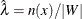.

The empty-space F function is defined as the empirical distribution function of the observed empty-space distances,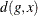, which is measured from a set of reference grid points g to the nearest point in the point pattern. The empty-space distance can be defined as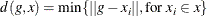In practice, the computation of the empty-space F function also involves an edge correction. The edge-corrected empty-space F function is defined as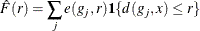where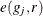is an edge correction. PROC SPP implements the border edge correction (Illian et al. 2008, p. 185–186) as described in the section Border Edge Correction for Distance Functions.

For a homogeneous Poisson process that has first-order intensity, the F function is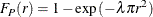You compare the empirical and Poisson empty-space F function by using the EDF and the P-P plot in the F function summary panel plot. Values of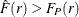suggest a regularly spaced pattern, and values of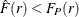suggest a clustered pattern (Baddeley and Turner 2005).

The nearest-neighbor G function is the empirical distribution of the observed nearest-neighbor distance of the points within the point pattern. In practice, the G function also involves an edge correction and is defined as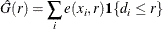where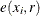is the border edge correction (Illian et al. 2008, p. 185-186) as described in the section Border Edge Correction for Distance Functions and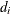is the distance to the nearest neighbor for the ith point.

For a homogeneous Poisson process that has first-order intensity, the G function can be defined as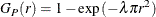The interpretation of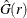is opposite to the interpretation of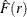. That is, values of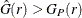imply a clustered pattern, and values of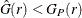suggest a regular pattern (Baddeley and Turner 2005).

The third type of nearest-neighbor distance function is the J function, which is defined as a combination of both the F and G functions (Baddeley et al. 2000). The J function is defined for all distances r such that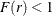. The J function can be defined as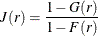For a homogeneous Poisson process,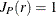. When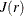takes values greater than 1, regularity is indicated; whentakes values less than 1, the underlying process is more clustered than expected. As can be seen from the expression of, the estimate is an uncorrected estimate of the J-function and hence its computation does not require an edge correction (Baddeley et al. 2000).